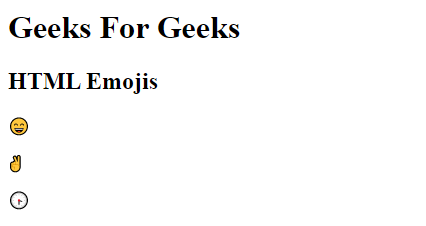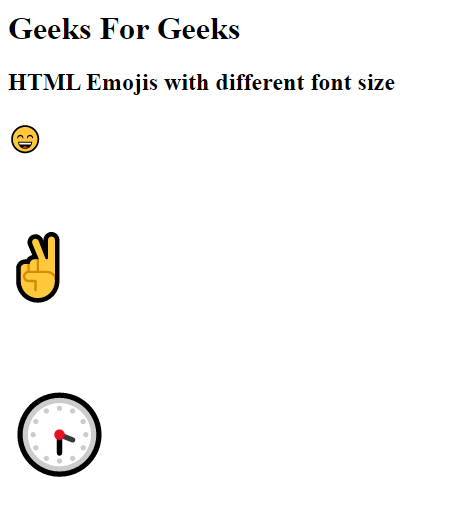# HTML Emojis

• Difficulty Level : Basic
• Last Updated : 27 Nov, 2020

Emojis are letters (characters) from the UTF-8 (Unicode) character set. The <meta charset=”UTF-8″> element in HTML defines the character set. Many UTF-8 characters cannot be typed on a keyboard, but they can always be displayed using numbers (called entity numbers). To let the browser understand that you are displaying a character, you must start the entity number with &# and end it with ; (semicolon).

Syntax:

```<head>
<meta charset="UTF-8">

<body>
<p>&#number;</p>

// Take 'number' corresponding
// to UTF-8 characters you want
// to display.
</body>
```

Emojis are also characters from the UTF-8 alphabet and can be used in HTML by mentioning the corresponding emoji, decimal (dec) or hexadecimal (hex) reference in the above syntax.

Example: Represent the following emoji in a webpage.

## HTML

 ` ` `<``html``> ` `<``head``> ` `    ``<``meta` `charset``=``"UTF-8"``> ` ` ` ` `  `<``body``> ` `    ``<``h1``>Geeks For Geeks ` `    ``<``h2``>HTML Emojis ` `    ``<``p``>😄 ` `    ``<``p``>✌ ` `    ``<``p``>🕞 ` ` ` ` `

Output:In the above example, the decimal reference used for the following emojis are as follows

Char Decimal reference Hexadecimal reference
😄 128516 1F604
9996 270C
🕞 128350 1F55E

Note: Instead of decimal (dec) reference, one can also use hexadecimal(hex)  reference to display emoji in a webpage. In addition to the above syntax, one need to include ‘x’ before hexadecimal reference.

Syntax:

```<p>&#xhexaDecimal;</p>
```

Example: In this example, we will represent 😄, ✌ and 🕞 emoji in a webpage with hexadecimal(hex) reference.

## HTML

 ` ` `<``html``> ` ` `  `<``head``> ` `    ``<``meta` `charset``=``"UTF-8"``> ` ` ` ` `  `<``body``> ` `    ``<``h1``>Geeks For Geeks ` `    ``<``h2``>HTML Emojis ` `    ``<``p``>😄 ` `    ``<``p``>✌ ` `    ``<``p``>🕞 ` ` ` ` `  ` `

Output:With hexadecimal reference also we are getting the same output.

Note: Since Emojis are characters, they can be copied, displayed and sized just like any other character in HTML.

Example: In this example, we will change the font size of the following emojis 😄, ✌ and 🕞.

## HTML

 ` ` `<``html``> ` ` `  `<``head``> ` `    ``<``meta` `charset``=``"UTF-8"``> ` ` ` ` `  `<``body``> ` `    ``<``h1``>Geeks For Geeks ` `    ``<``h2``>HTML Emojis with different font size ` `    ``<``p` `style``=``"font-size:25px"``>😄 ` `    ``<``p` `style``=``"font-size:65px"``>✌ ` `    ``<``p` `style``=``"font-size:75px"``>🕞 ` ` ` ` `  ` `

Output: The output contains the different sizes of emojis.Similarly any emoji can be displayed in a webpage by entering the corresponding decimal or hexadecimal reference in a code.

My Personal Notes arrow_drop_up
Recommended Articles
Page :